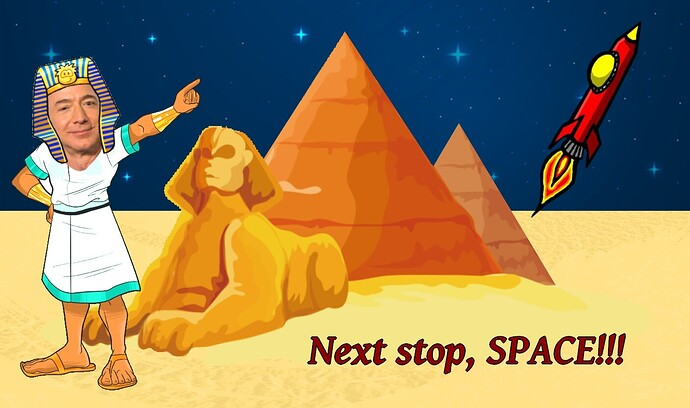-=+=- -=+=- -=+=- -=+=- -=+=- -=+=- -=+=- -=+=- -=+=- -=+=- -=+=- -=+=- -=+=- -=+=- -=+=- -=+=- -=+=- -=+=- -=+=- -=+=- -=+=- -=+=- -=+=- -=+=- -=+=- -=+=- -=+=- -=+=- -=+=- -=+=- (c) WidthPadding Industries 1987 0|49|0 -=+=- -=+=- -=+=- -=+=- -=+=- -=+=- -=+=- -=+=- -=+=- -=+=- -=+=- -=+=- -=+=- -=+=- -=+=- -=+=- -=+=- -=+=- -=+=- -=+=- -=+=- -=+=- -=+=- -=+=- -=+=- -=+=- -=+=- -=+=- -=+=- -=+=-
Socoder -> Off Topic -> Branson Goes to Space

Posted : Sunday, 11 July 2021, 11:04
Jayenkai### Branson Goes to SpacePosted : Sunday, 11 July 2021, 12:42
PHSYeah but did he really?
Posted : Tuesday, 20 July 2021, 07:44
JayenkaiBezosesieses turn.

-=-=-Posted : Tuesday, 20 July 2021, 09:15
PHSLooks fake.
Posted : Tuesday, 20 July 2021, 09:24
PHSPosted : Tuesday, 20 July 2021, 14:47
Jayenkai